# Data#

## Datasets#

### data()#

data(dataset='bio_eventrelated_100hz')[source]#

NeuroKit Datasets

NeuroKit includes datasets that can be used for testing. These datasets are not downloaded automatically with the package (to avoid increasing its weight), but can be downloaded via the `nk.data()` function (note that an internet connection is necessary). See the examples below.

Signals: The following signals (that will return an array) are available:

• ecg_1000hz: Returns a vector containing ECG signal (`sampling_rate=1000`).

• ecg_3000hz: Returns a vector containing ECG signal (`sampling_rate=3000`).

• rsp_1000hz: Returns a vector containing RSP signal (`sampling_rate=1000`).

• eeg_150hz: Returns a vector containing EEG signal (`sampling_rate=150`).

• eog_100hz: Returns a vector containing vEOG signal (`sampling_rate=100`).

DataFrames: The following datasets (that will return a `pd.DataFrame`) are available:

• iris: Convenient access to the Iris dataset in a DataFrame, exactly how it is in R.

• eogs_200hz: Returns a DataFrame with `hEOG`, `vEOG`.

• Single subject

• Visual and horizontal electrooculagraphy

• `sampling_rate=200`

• bio_resting_5min_100hz: Returns a DataFrame with `ECG`, `PPG`, `RSP`.

• Single subject

• Resting-state of 5 min (pre-cropped, with some ECG noise towards the end)

• `sampling_rate=100`

• bio_resting_8min_100hz: Returns a DataFrame with `ECG`, `RSP`, `EDA`, `PhotoSensor`.

• Single subject

• Resting-state of 8 min when the Photosensor is low (need to crop the data)

• `sampling_rate=100`

• bio_resting_8min_200hz: Returns a dictionary with four subjects (`S01`, `S02`, `S03`, `S04`).

• Resting-state recordings

• 8 min (`sampling_rate=200`)

• Each subject is DataFrame with `ECG`, `RSP`, ``PhotoSensor`, `Participant`

• bio_eventrelated_100hz: Returns a DataFrame with `ECG`, `EDA`, `Photosensor`, `RSP`.

• Single subject

• Event-related recording of a participant watching 4 images for 3 seconds (the condition order was: `["Negative", "Neutral", "Neutral", "Negative"]`)

• `sampling_rate=100`

Parameters

dataset (str) – The name of the dataset.

Returns

DataFrame – The data.

Examples

Single signals and vectors

```In : import neurokit2 as nk

In : ecg = nk.data(dataset="ecg_1000hz")

In : nk.signal_plot(ecg[0:10000], sampling_rate=1000)
```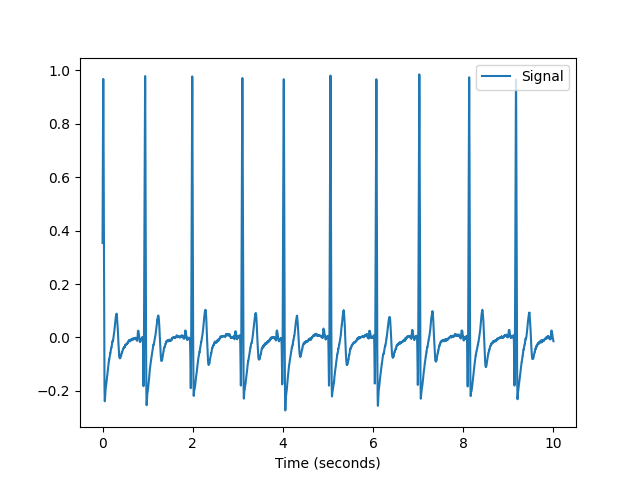```In : rsp = nk.data(dataset="rsp_1000hz")

In : nk.signal_plot(rsp[0:20000], sampling_rate=1000)
```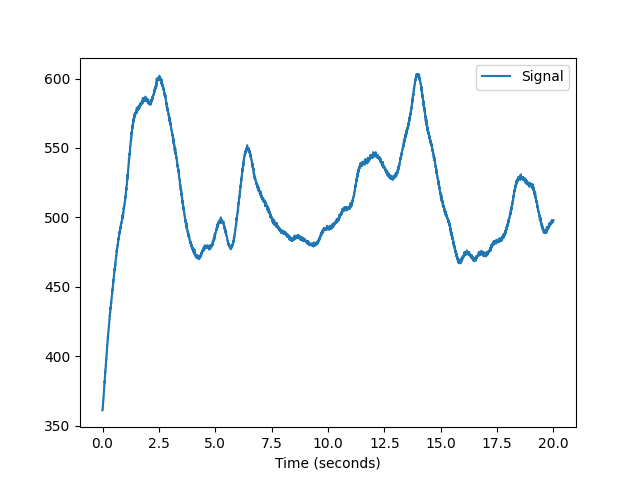```In : eeg = nk.data("eeg_150hz")

In : nk.signal_plot(eeg, sampling_rate=150)
```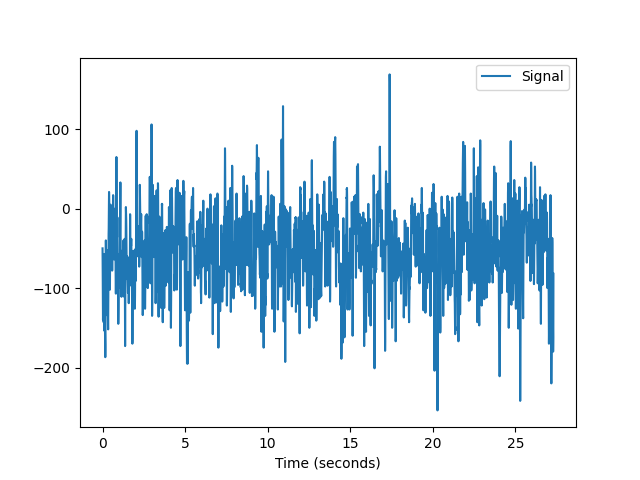```In : eog = nk.data("eog_100hz")

In : nk.signal_plot(eog[0:2000], sampling_rate=100)
```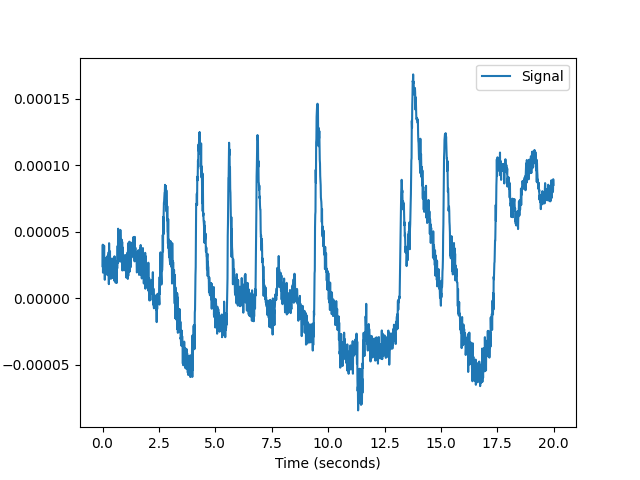DataFrames

```In : data = nk.data("iris")

Out:
Sepal.Length  Sepal.Width  Petal.Length  Petal.Width Species
0           5.1          3.5           1.4          0.2  setosa
1           4.9          3.0           1.4          0.2  setosa
2           4.7          3.2           1.3          0.2  setosa
3           4.6          3.1           1.5          0.2  setosa
4           5.0          3.6           1.4          0.2  setosa
```
```In : data = nk.data(dataset="eogs_200hz")

In : nk.signal_plot(data[0:4000], standardize=True, sampling_rate=200)
```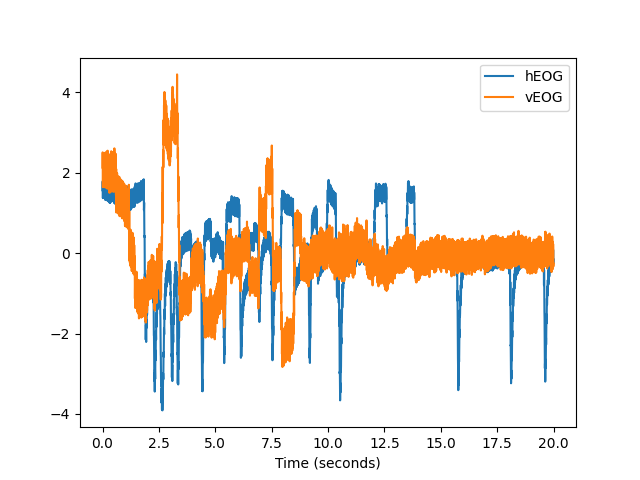```In : data = nk.data(dataset="bio_resting_5min_100hz")

In : nk.standardize(data).plot()
Out: <AxesSubplot:>
```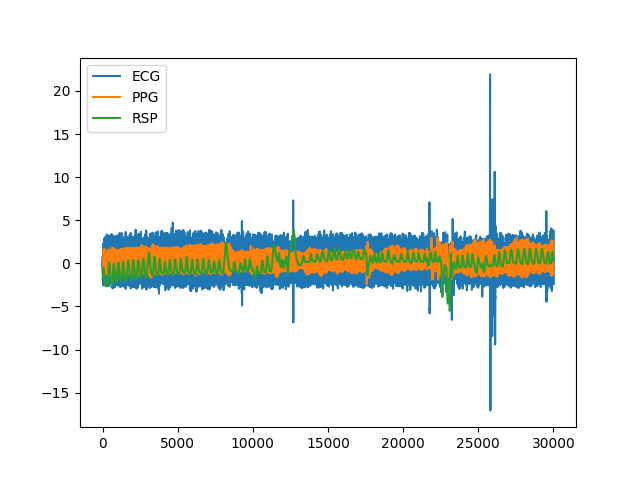```In : data = nk.data(dataset="bio_resting_8min_100hz")

In : nk.standardize(data).plot()
Out: <AxesSubplot:>
```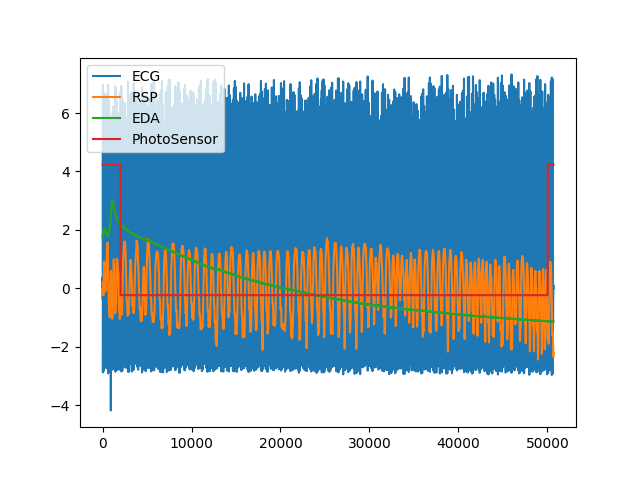```In : data = nk.data("bio_resting_8min_200hz")

In : data.keys()
Out: dict_keys(['S01', 'S02', 'S03', 'S04'])

Out:
ECG       RSP  PhotoSensor Participant
0  2.394536e-19  5.010681          5.0         S01
1  1.281743e-02  5.011291          5.0         S01
2  1.129138e-02  5.010376          5.0         S01
3  7.629118e-04  5.010681          5.0         S01
4 -4.119742e-03  5.010986          5.0         S01
```
```In : data = nk.data("bio_eventrelated_100hz")

In : nk.standardize(data).plot()
Out: <AxesSubplot:>
```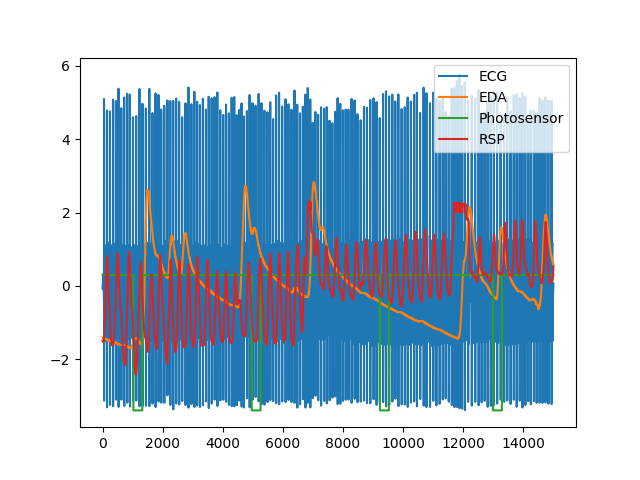## I/O#

Read and format a BIOPAC’s AcqKnowledge file into a pandas’ dataframe

The function outputs both the dataframe and the sampling rate (retrieved from the AcqKnowledge file).

Parameters
• filename (str) – Filename (with or without the extension) of a BIOPAC’s AcqKnowledge file (e.g., `"data.acq"`).

• sampling_rate (int) – Sampling rate (in Hz, i.e., samples/second). Since an AcqKnowledge file can contain signals recorded at different rates, harmonization is necessary in order to convert it to a DataFrame. Thus, if sampling_rate is set to `max` (default), will keep the maximum recorded sampling rate and upsample the channels with lower rate if necessary (using the `signal_resample()` function). If the sampling rate is set to a given value, will resample the signals to the desired value. Note that the value of the sampling rate is outputted along with the data.

• resample_method (str) – Method of resampling (see `signal_resample()`).

• impute_missing (bool) – Sometimes, due to connections issues, there are lapses in the recorded signal (short periods without signal). If `impute_missing` is `True`, will automatically fill the signal interruptions using padding.

Returns

• df (DataFrame) – The AcqKnowledge file as a pandas dataframe.

• sampling rate (int) – The sampling rate at which the data is sampled.

Example

```In : import neurokit2 as nk

```

Read and format a OpenSignals file (e.g., from BITalino) into a pandas’ dataframe

The function outputs both the dataframe and the sampling rate (retrieved from the OpenSignals file).

Parameters
• filename (str) – Filename (with or without the extension) of an OpenSignals file (e.g., `"data.txt"`).

• sampling_rate (int) – Sampling rate (in Hz, i.e., samples/second). Defaults to the original sampling rate at which signals were sampled if set to `max`. If the sampling rate is set to a given value, will resample the signals to the desired value. Note that the value of the sampling rate is outputted along with the data.

• resample_method (str) – Method of resampling (see `signal_resample()`).

• events_annotation (bool) – Defaults to False. If True, will read signal annotation events.

• events_annotation_directory (str) – If `None` (default), reads signal annotation events from the same location where the acquired file is stored. If not, specify the predefined OpenSignals (r)evolution folder directory of where the `"EventsAnnotation.txt"` file is stored.

Returns

• df (DataFrame, dict) – The BITalino file as a pandas dataframe if one device was read, or a dictionary of pandas dataframes (one dataframe per device) if multiple devices are read.

• info (dict) – The metadata information containing the sensors, corresponding channel names, sampling rate, and the events annotation timings if `events_annotation` is `True`.

Examples

```In : import neurokit2 as nk

```

### write_csv()#

write_csv(data, filename, parts=None, **kwargs)[source]#

Write data to multiple csv files

Split the data into multiple CSV files. You can then re-create them as follows:

Parameters
• data (list) – List of dictionaries.

• filename (str) – Name of the CSV file (without the extension).

• parts (int) – Number of parts to split the data into.

Returns

None

Example

Save big file in parts

```In : import pandas as pd

In : import neurokit2 as nk

# Split data into multiple files
# nk.write_csv(data, 'C:/Users/.../data', parts=6)
```

```# Iterate through 6-parts and concatenate the pieces